# Quadratics in Vertex Form Objective Students will write

• Slides: 15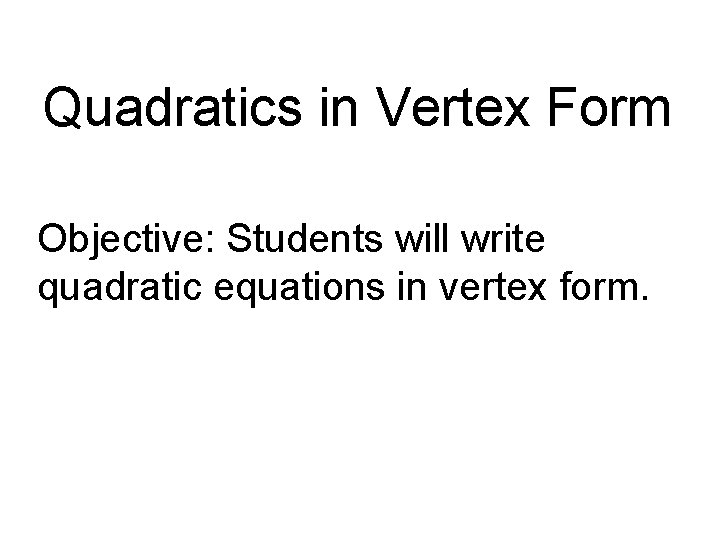Quadratics in Vertex Form Objective: Students will write quadratic equations in vertex form.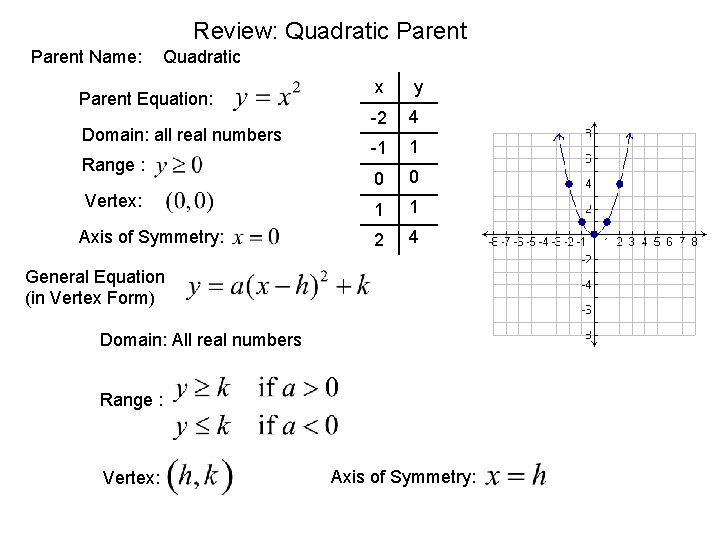Review: Quadratic Parent Name: Quadratic Parent Equation: Domain: all real numbers Range : Vertex: Axis of Symmetry: x y -2 4 -1 1 0 0 1 1 2 4 General Equation (in Vertex Form) Domain: All real numbers Range : Vertex: Axis of Symmetry: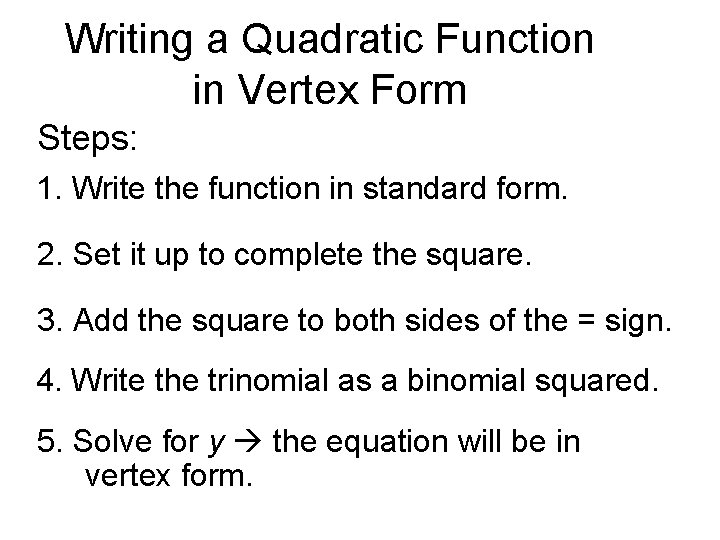Writing a Quadratic Function in Vertex Form Steps: 1. Write the function in standard form. 2. Set it up to complete the square. 3. Add the square to both sides of the = sign. 4. Write the trinomial as a binomial squared. 5. Solve for y the equation will be in vertex form.Writing a Quadratic Function in Vertex Form Example 1: Write the function in vertex form and identify its vertex. 1. Write the function in standard form. 2. Set it up to complete the square.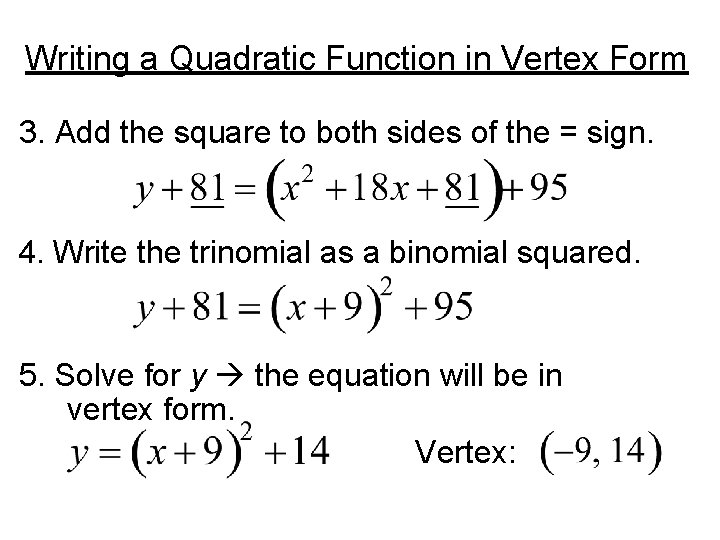Writing a Quadratic Function in Vertex Form 3. Add the square to both sides of the = sign. 4. Write the trinomial as a binomial squared. 5. Solve for y the equation will be in vertex form. Vertex:Practice: Write a quadratic function in vertex form and identify its vertex. P 1: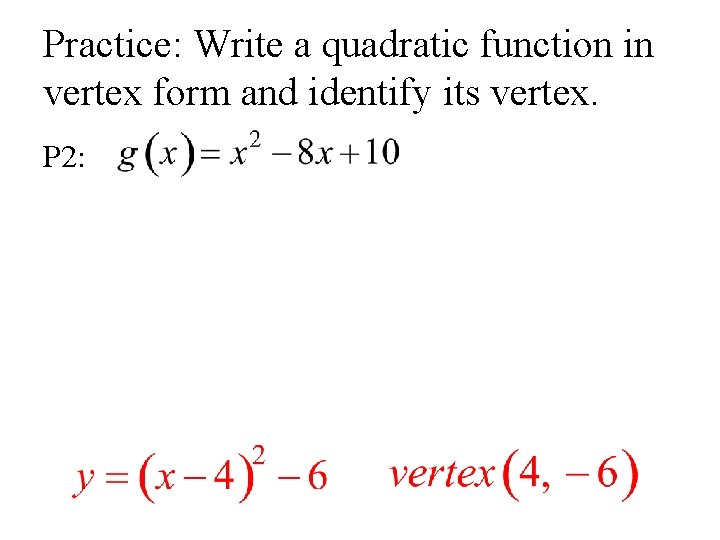Practice: Write a quadratic function in vertex form and identify its vertex. P 2: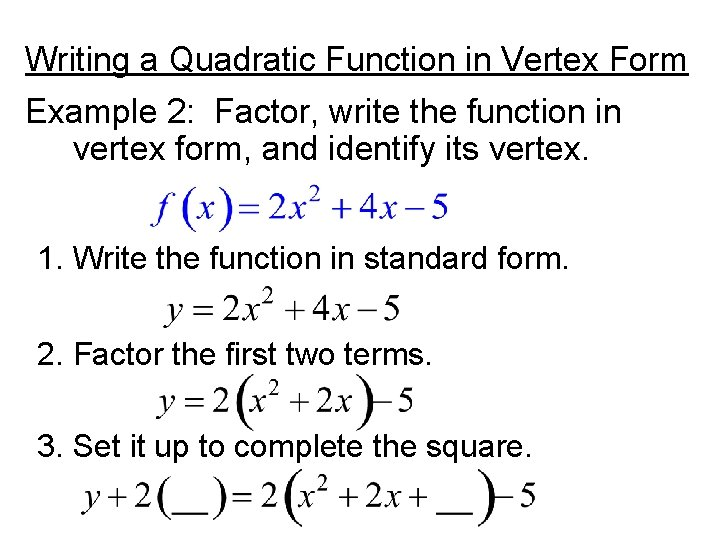Writing a Quadratic Function in Vertex Form Example 2: Factor, write the function in vertex form, and identify its vertex. 1. Write the function in standard form. 2. Factor the first two terms. 3. Set it up to complete the square.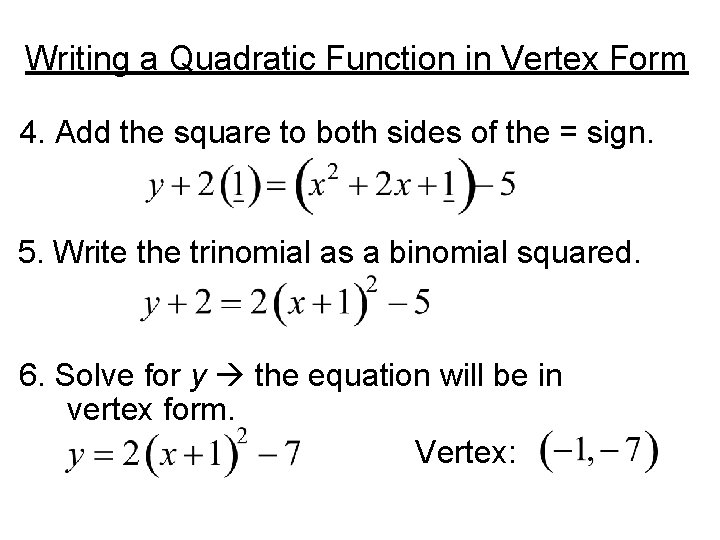Writing a Quadratic Function in Vertex Form 4. Add the square to both sides of the = sign. 5. Write the trinomial as a binomial squared. 6. Solve for y the equation will be in vertex form. Vertex: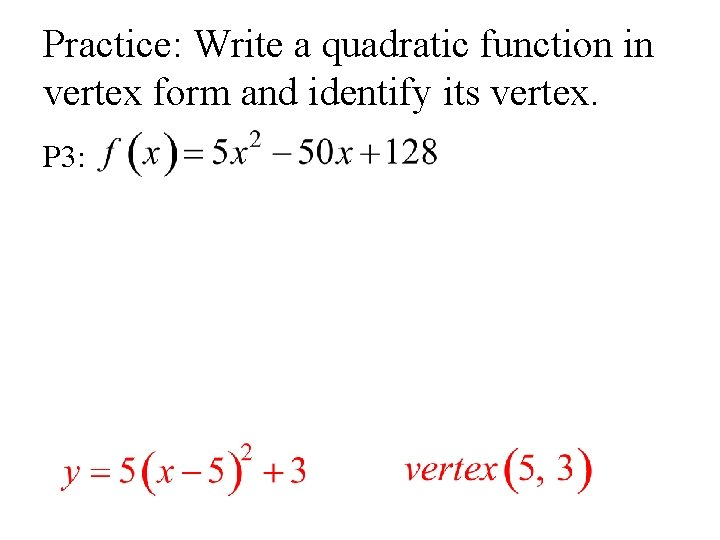Practice: Write a quadratic function in vertex form and identify its vertex. P 3: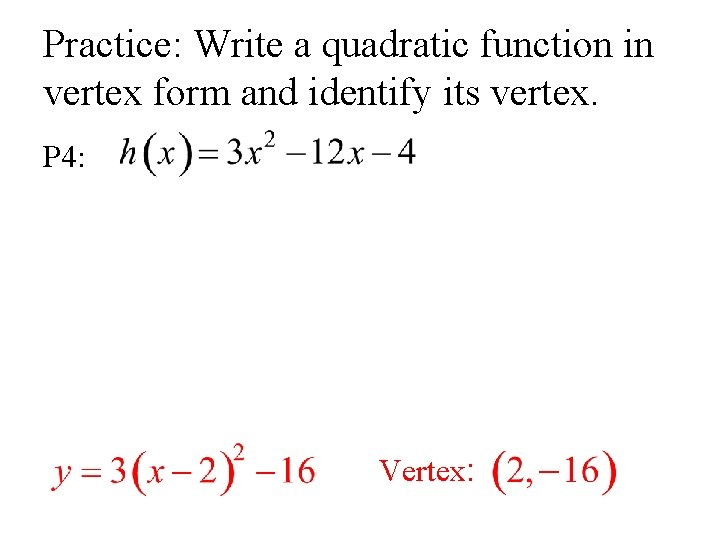Practice: Write a quadratic function in vertex form and identify its vertex. P 4: Vertex:Writing a Quadratic Function in Vertex Form Example 3: Write the function, using fractions, in vertex form, and identify its vertex. 1. Write the function in standard form. 2. Set it up to complete the square.Writing a Quadratic Function in Vertex Form 3. Add the square to both sides of the = sign. 4. Write the trinomial as a binomial squared. 5. Solve for y the equation will be in vertex form. Vertex:Practice: Write a quadratic function in vertex form and identify its vertex. P 5: Vertex: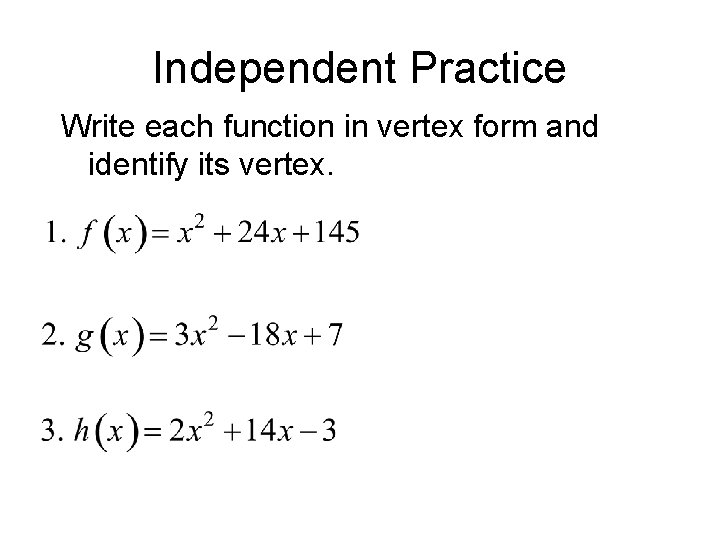Independent Practice Write each function in vertex form and identify its vertex.